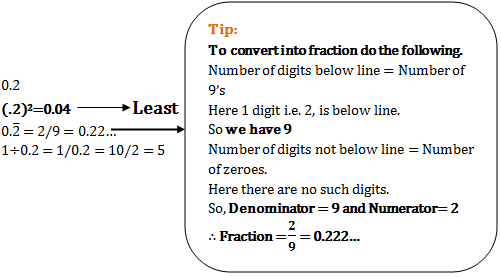# Aptitude Placement Test for Engineering Students - Set 3

1)   The marked price of a watch is Rs. 800. A shopkeeper gives two successive discounts and sells the watch at Rs. 612. If the first discount is 10%, the second discount is

a. 12%
b. 20%
c. 15%
d. 10%
 Answer  Explanation ANSWER: 15% Explanation: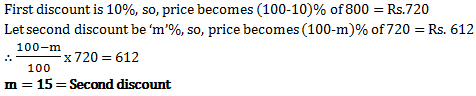2)   A train moves with the speed of 180 km/hr. Its speed (in metres per second) is

a. 5
b. 40
c. 30
d. 50
 Answer  Explanation ANSWER: 50 Explanation: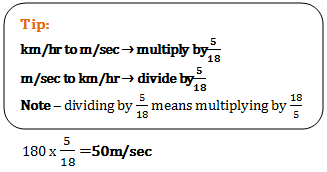3)   A car travelling with 5/7th of its actual speed covers 42km in 1hr. 40mins. 48 secs. Find the actual speed of the car.

a. 17(6/7) km/hr
b. 35 km/hr
c. 25 km/hr
d. 30 km/hr
 Answer  Explanation ANSWER: 35 km/hr Explanation: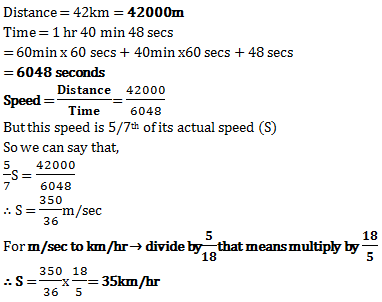4)   A train 120 m long passes a telegraph post in 6 seconds. Find the speed of the train.

a. 60 km/hr
b. 72.5 km/hr
c. 80 km/hr
d. 72 km/hr
 Answer  Explanation ANSWER: 72 km/hr Explanation: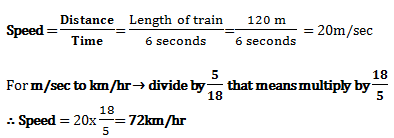5)   A train passes a telegraph post in 8 seconds and a 264 m long bridge in 20 seconds. What is the length of the train

a. 180 m
b. 176 m
c. 164 m
d. 158 m
 Answer  Explanation ANSWER: 176 m Explanation: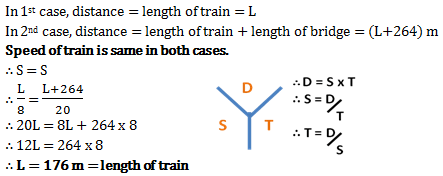6)   If ratio of the areas of two squares is 1:4, the ratio of their perimeters is

a. 1:2
b. 1:4
c. 1:6
d. 1:8
 Answer  Explanation ANSWER: 1:2 Explanation: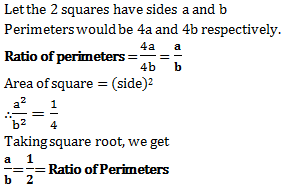7)   The perimeter of a square is 48cm. The area of rectangle is 4cm2 less than the area of the square. If length of the rectangle is 14cm, then its perimeter is

a. 24cm
b. 48cm
c. 50cm
d. 54cm
 Answer  Explanation ANSWER: 48cm Explanation: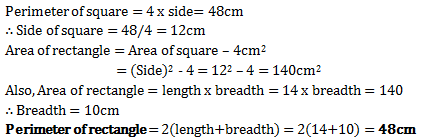8)   An equilateral triangle is described on the diagonal of a square. What is the ratio of the area of the triangle to that of the square?

a. 3:2
b. 2:3
c. 3:4
d. 4:3
 Answer  Explanation ANSWER: 3:2 Explanation: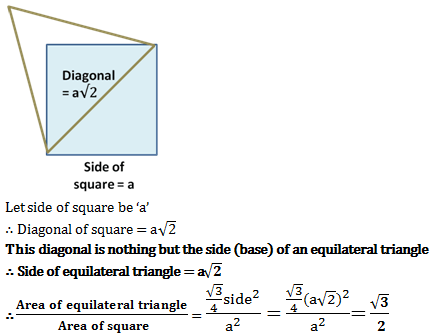9)   The side and one of the diagonals of a rhombus are 13cm and 24cm respectively. The area of the rhombus (in sq.cm.) is

a. 156
b. 240
c. 120
d. 130
 Answer  Explanation ANSWER: 120 Explanation: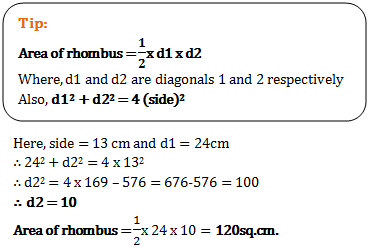10)   A room is 6m long, 5m broad and 4m high. The maximum length of rod that can be kept in the room is

a. 61m
b. 16m
c. 36m
d. 77m
 Answer  Explanation ANSWER: 77m Explanation: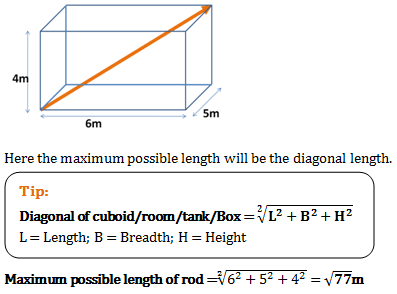11)   Average age of A and B is 30 years, that of B and C is 32 years and the average age of C and A is 34 years. The age of C is

a. 33 years
b. 34 years
c. 35 years
d. 36 years
 Answer  Explanation ANSWER: 36 years Explanation: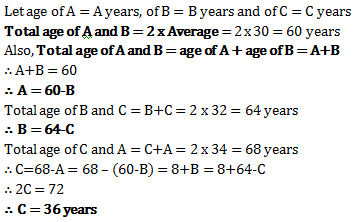12)   The average of three numbers is 77. The first number is twice the second and the second number is twice the third. Find the first number.

a. 33
b. 66
c. 77
d. 132
 Answer  Explanation ANSWER: 132 Explanation: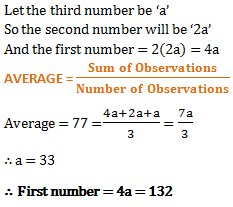13)   The average of fifty numbers is 28. If two numbers, namely 25 and 35 are discarded, the average of the remaining numbers is nearly,

a. 29.27
b. 27.92
c. 27.29
d. 29.72
 Answer  Explanation ANSWER: 27.92 Explanation: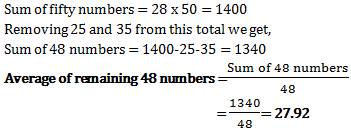14)   If square root of 5625 is 75, then 5625 + 56.25 + 0.5625 is equal to

a. 9
b. 83.25
c. 82.80
d. 8.325
 Answer  Explanation ANSWER: 83.25 Explanation: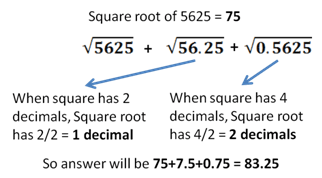15)   461 + 462 + 463 + 464 + 465 is divisible by

a. 3
b. 5
c. 11
d. 17
 Answer  Explanation ANSWER: 11 Explanation: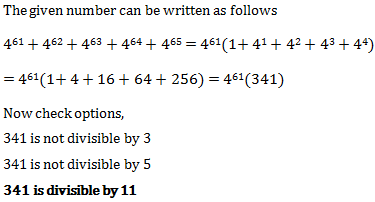16)   What is value of
 .1 x .1 x .1 + .02 x .02 x .02 .2 x .2 x .2 +  .04 x .04 x .04

a. 0.0125
b. 0.125
c. 0.25
d. 0.5
 Answer  Explanation ANSWER: 0.125 Explanation: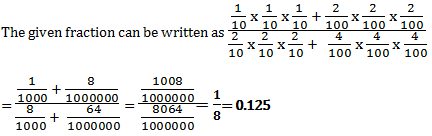17)   If a = 4.36, b = 2.39, c = 1.97, then the value of a3-b3-c3-3abc is

a. 3.94
b. 2.39
c. 0
d. 1
 Answer  Explanation ANSWER: 0 Explanation: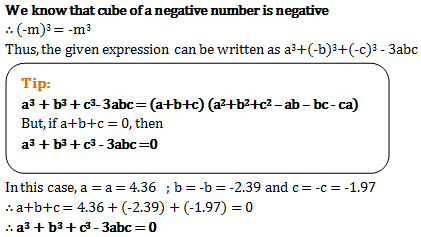18)   If a*b = 2a+3b, then the value of 2*3 + 3*4 is

a. 24
b. 31
c. 32
d. 34
 Answer  Explanation ANSWER: 31 Explanation: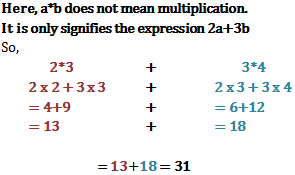19)   If 12+22+32+…..+ 102 = 385, then 32+62+92+….+302 is equal to

a. 3465
b. 2310
c. 1155
d. 770
 Answer  Explanation ANSWER: 3465 Explanation: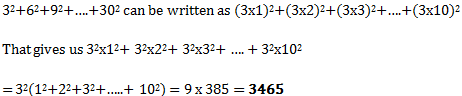20)   The least of the following 0.2, (.2)2, 0.2, 1÷0.2

a. (.2)2
b. 0.2
c. 0.2
d. 1÷0.2
 Answer  Explanation ANSWER: (.2)2 Explanation: